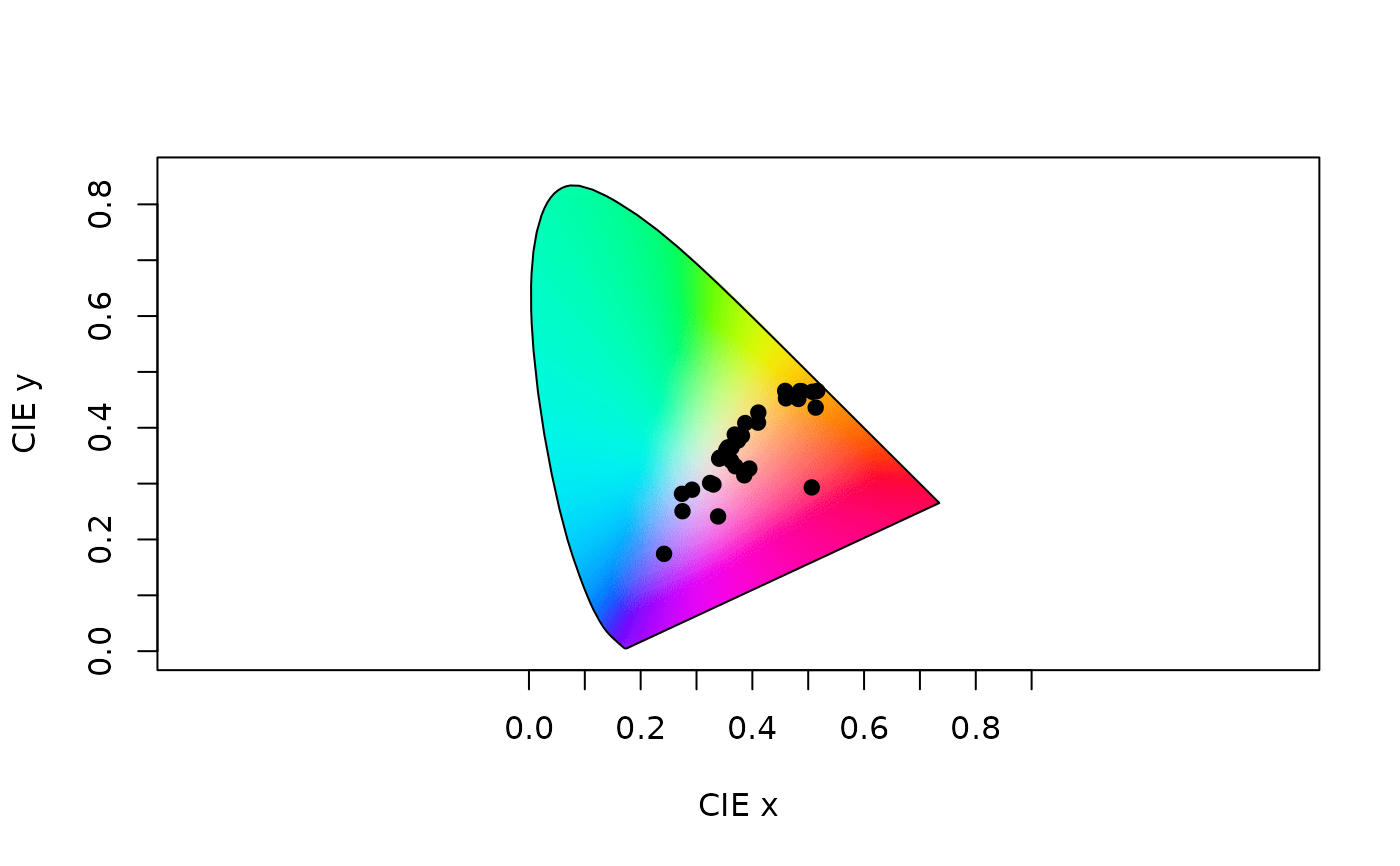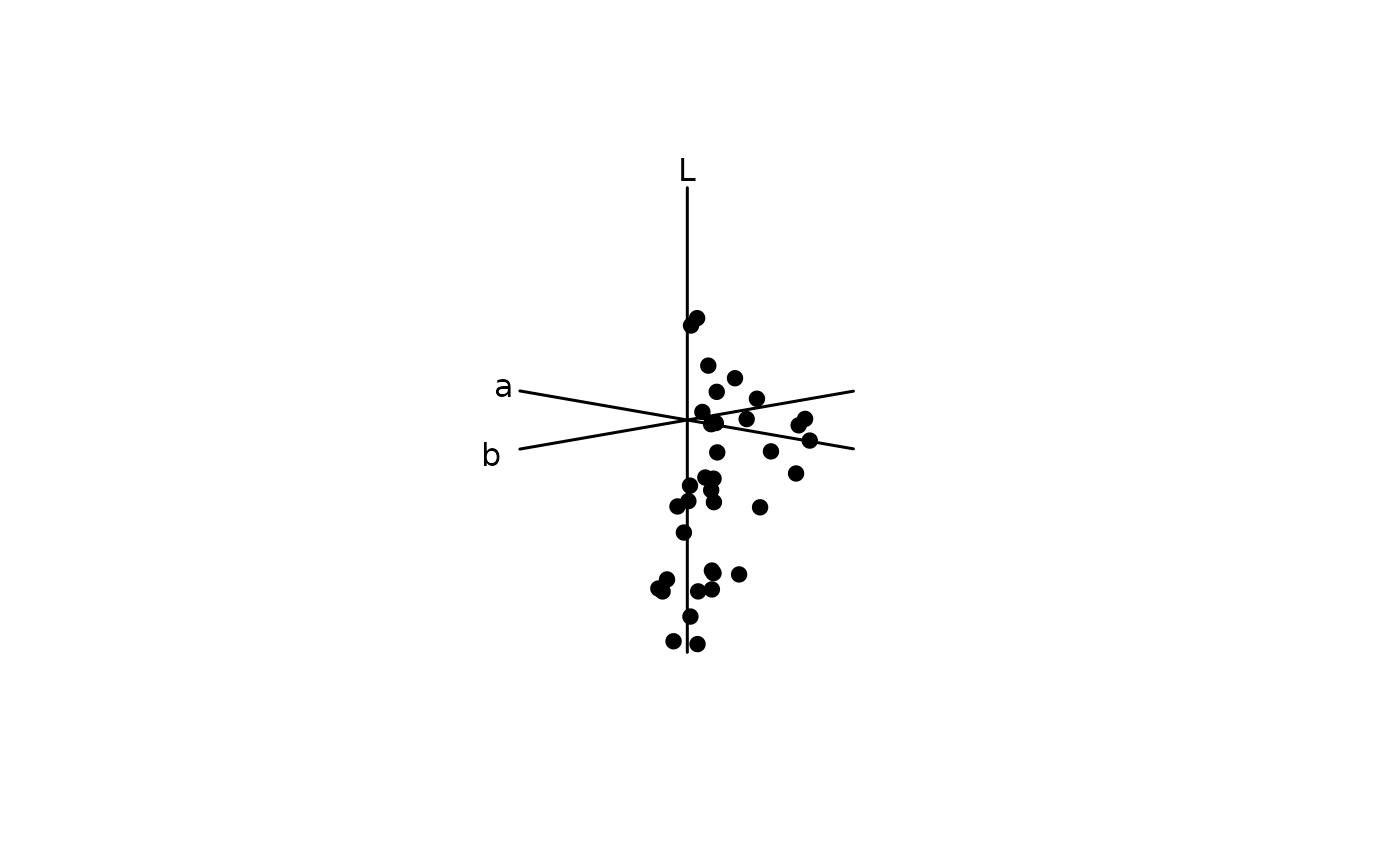Plot a CIE (XYZ, LAB, or LCH) chromaticity diagram.

cieplot(
ciedata,
mono = TRUE,
out.lwd = NULL,
out.lcol = "black",
out.lty = 1,
theta = 45,
phi = 10,
r = 1e+06,
zoom = 1,
box = FALSE,
ciebg = TRUE,
...
)

## Arguments

ciedata

(required). a data frame, possibly a result from the colspace() or cie() function, containing values for 'x', 'y' and 'z' coordinates for the CIEXYZ model, or LAB coordinates for the CIELAB (or CIELCh models), as columns (labeled as such).

mono

should the monochromatic loci (the 'horseshoe') be plotted when space = "ciexyz"? Defaults to TRUE.

out.lwd, out.lcol, out.lty

graphical parameters for the plot outline.

theta

angle to rotate the plot in the xy plane when space = "cielab" (defaults to 10).

phi

angle to rotate the plot in the yz plane when space = "cielab" (defaults to 45).

r

the distance of the eyepoint from the center of the plotting box when space = "cielab". Very high values approximate an orthographic projection (defaults to 1e6). See graphics::persp() for details.

zoom

zooms in (values greater than 1) or out (values between 0 and 1) from the plotting area when space = "cielab".

box

logical. Should the plot area box and axes be plotted? (defaults to FALSE)

ciebg

should the colour background be plotted for CIEXYZ plot? (defaults to TRUE)

...

additional graphical options. See par().

## Author

Thomas E. White thomas.white026@gmail.com

Rafael Maia rm72@zips.uakron.edu

## Examples

# Load floral reflectance spectra
data(flowers)

# CIEXYZ
# Estimate quantum catches, using the cie10-degree viewer matching function
vis.flowers <- vismodel(flowers, visual = "cie10", illum = "D65", vonkries = TRUE, relative = FALSE)

# Run the ciexyz model
xyz.flowers <- colspace(vis.flowers, space = "ciexyz")

# Visualise the floral spectra in a ciexyz chromaticity diagram
plot(xyz.flowers)# CIELAB
# Using the quantum catches above, instead model the spectra in the CIELab
# space
lab.flowers <- colspace(vis.flowers, space = "cielab")

# And plot in Lab space
plot(lab.flowers)